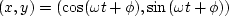Next: Superposition of sinusoids Up: Convolution and Spectra Previous: Negative time

# FOURIER SUMS

The world is filled with sines and cosines. The coordinates of a point on a spinning wheel are,whereis the angular frequency of revolution andis the phase angle. The purest tones and the purest colors are sinusoidal. The movement of a pendulum is nearly sinusoidal, the approximation going to perfection in the limit of small amplitude motions. The sum of all the tones in any signal is its spectrum.''

Small amplitude signals are widespread in nature, from the vibrations of atoms to the sound vibrations we create and observe in the earth. Sound typically compresses air by a volume fraction of 10-3 to 10-6. In water or solid, the compression is typically 10-6 to 10-9. A mathematical reason why sinusoids are so common in nature is that laws of nature are typically expressible as partial differential equations. Whenever the coefficients of the differentials (which are functions of material properties) are constant in time and space, the equations have exponential and sinusoidal solutions that correspond to waves propagating in all directions.Next: Superposition of sinusoids Up: Convolution and Spectra Previous: Negative time
Stanford Exploration Project
10/21/1998